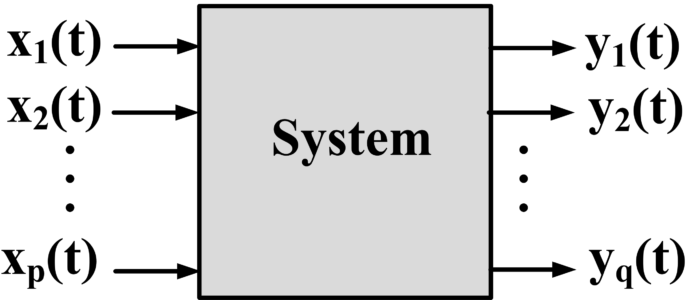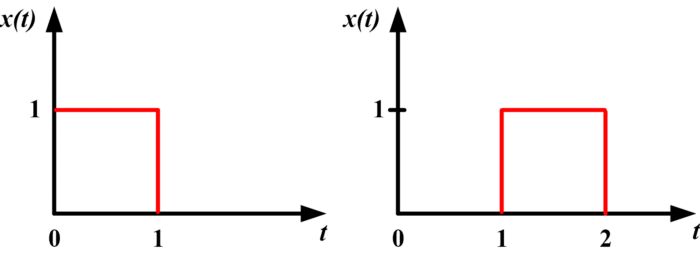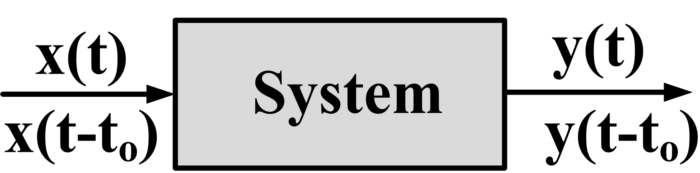# Basic System Properties

Want create site? Find Free WordPress Themes and plugins.

## Definition of a System

A common way of viewing a system is in terms of a “black box” with terminals, as illustrated in the following figure:In the figure, x1(t), x2(t)… xp(t) are the signals applied to the p input terminals of the system and y1 (t), y2 (t)… yq (t) are the resulting responses appearing at the q output terminals of the system.

In general, p is not equal to q; in other words, the number of input terminals may not equal the number of output terminals. When p=q=1, the system is a signal–input single–outputs system, sometimes referred to as a scalar system.

## Continuous-time system

When the time variable t takes its values from the set of real numbers, the system is said to be a continuous time system or and an analog system.In the above figure, both x (t) and y (t) are continuous time input and output respectively.

## Discrete time system

When the time variable t takes on the discrete values t=kT, k=…,-2,-1,0,1,2,… the system is said to be discrete – time system.In above figure, input x[n] and output y[n] are discrete in nature.

A digital system is a discrete –time system whose inputs and outputs are digital signals; That is, the input and output signals can take on only finite number of different values (e.g. 0 or 1)

A common type of continuous-time signal is a voltage or current waveform in an RLC or in an integrated circuit. Other common types of continuous- time signals are force and torque applied to a mechanical device, and angular positions or angular velocities of a rotor in a DC motor.

Discrete-time signals arise naturally in many areas of business, science, and engineering. One of the most common ways in which discrete-time signals arise is in sampling continuous –time signals.

The extent to which a system can be studied using analytical techniques depends on the properties of the system. There are certain principal properties that numerous systems share whether they are continuous time or discrete- time in nature. A comprehension of these crucial properties permits a designer to devise tools which can be used to for the analysis of a particular system.

In this section, the following properties are defined in detail:

1. Causality
2. Linearity
3. Time invariance
4. Memoryless system and system with memory
5. System invertibility

## Causality

A system is causal if its output at a time t does not rely on input x (t) for t >t1. It means that present output does not depend on future input, but only present and past values of input.

Suppose that the system is a continuous time system given by

y (t) = x(t-1)

This system is causal since the values of the output at time t depends on only the value of the input at time t-1.

if we apply the pulse shown in the following figure (left) to this system, the output is the pulse shown in the figure (right).From above figure, we see that the system delays the input pulse by 1 second.

## Linearity

The system is linear if it is both additive and homogeneous

A system F is said to be additive if

For any two inputs x and  .

In other words, the system is additive if the output response resulting from the input   is equal to the output response resulting from x plus the output response resulting from  .

Homogeneous

The system F is said to be homogeneous If

For any input x and scalar a.

By above, the system is homogeneous if the output response resulting from the input ax is equal to a times the output response resulting from x.

It is easier to see that the conditions (1) and (2) are equivalent to the single condition given below:

For all inputs x and  and scalars a and b.

Hence the given system is linear if and only if condition (3) is satisfied. By (3), the system is linear if for any inputs x and  and any scalars a,b, the output response resulting from the input ax+b is equal to a times the repose to x plus b times the response to .

Examples of linear system

Scaling system, differentiator, integrator, time shift, convolution, difference system

## Time Invariance

In a time-invariant system, there are no changes in system structure as a function of a time t. A system is time-invariant if:From above, we can observe that for any time to and any input x (t) that produces response y (t), the response to the time-shifted input x (t-to) is equal to the time-shifted output y (t-to) of y (t).

Therefore, in a time-invariant system, the response to a left or right shift of the input x (t) is equal to a corresponding left or right shift in the response y (t).

Example of Time-Invariant Systems

An electric circuit with fixed values of Resistance R, inductance L, and capacitance C can be considered as time invariant.

## System with memory

A system has memory if the output at time t depends on future or past values of the input x (t).

Example

In RLC electric circuits, the energy stored in inductor and capacitor at time t depends on the input parameters for certain range of values of time t.

## Memoryless system

A causal system is memoryless if for any time t, the value of the output at time t depends only on the value of the input at time t.

Example

Suppose that y(t)=kx(t)where k is a fixed real number. At any time t1

y (t1)=k x(t1)

And thus y (t1) depends only on the values of the input at time t1 Hence the system is memoryless.

## System Invertibility

Invertibility in the system comes from a basic mathematical phenomenon called “inverse”. If input signal can be retrieved from output signal then we say that particular system is invertible.

Example

Let’s say, we have the following system;So, we will have;

y= Fx

For inversion purpose, we have Finv, such that

x=Finv y

Putting value of y in above expression, we obtain the following

x=Finv F x

Since,

Finv F =I= Identity Matrix

So,Did you find apk for android? You can find new Free Android Games and apps.

### 1 thought on “Basic System Properties”

1.Amazing Article.I never saw such a comprehensive yet easy to understand tutorial.Keep it up.